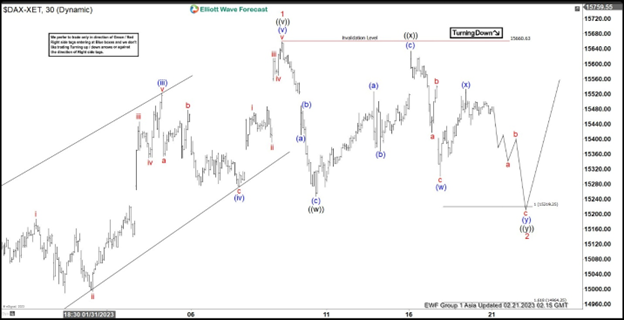Share:

Since forming the low on September 2022 at 11862.84, DAX shows an incomplete higher high (bullish) sequence. This favors further upside against 12.20.2022 low at 13791.52. Cycle from 12.20.2022 low ended as an impulsive Elliott Wave structure with wave 1 at 15660.63, as the 30 minutes chart below shows. Pullback in wave 2 is currently in progress to correct cycle from 12.20.2022 low. Structure of the pullback takes the form of a double three Elliott Wave structure.  Down from wave 1, wave (a) ended at 15410.82, wave (b) ended at 15475.05, and wave (c) lower ended at 15246.39 which completed wave ((w)).

## DAX 30 Minutes hour Elliott Wave chartRally in wave ((x)) ended at 15634.04 with subdivision as a zigzag Elliott Wave structure. Up from wave ((w)), wave (a) ended at 15526.28, pullback in wave (b) ended at 15366.37, and wave (c) higher ended at 15634.04 which completed wave ((x)) in higher degree. Wave ((y)) lower is now in progress with internal subdivision as another double three in lesser degree. Down from wave ((x)), wave (w) ended at 15300.42 and rally in wave (x) ended at 15532.44. Near term, as far as Index stays below 15660.63, expect the Index to extend lower to reach 14964.25 – 15219.25. This is the support zone for the Index, which is the 100% – 161.8% Fibonacci extension of wave ((w)). From this area, DAX can extend higher or rally in 3 waves at least.

### DAX Elliott Wave video

[embedded content]